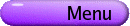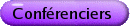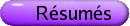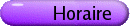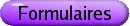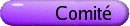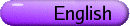Systèmes dynamiques
Org: Sue Ann Campbell (Waterloo), Yuming Chen (Wilfrid Laurier) et Huaiping Zhu (York)
[PDF]

JULIEN ARINO, McMaster University, 1280 Main Street West, Hamilton, Ontario L8S 4K1
Mathematical aspects of metapopulation disease models
[PDF]

Metapopulation disease models describe the spread of an infectious disease in a population that is, typically, spatially fragmented. Such models consist of systems of differential equations that are embedded in graphs. The resulting large dimensionality renders their mathematical analysis difficult. I will present some of the problems that arise when dealing with this type of models, and some of the solutions that were proposed to these problems.

CHRIS BAUCH, University of Guelph, 50 Stone Road East, Guelph, ON N1G 2W1
Dynamic games with imitation predict vaccinating behaviour
[PDF]

There exists an interplay between vaccine coverage, disease prevalence, and the vaccinating behaviour of individuals. Moreover, because of herd immunity, there is also a strategic interaction between individuals when they are deciding whether or not to vaccinate, since the probability that an individual becomes infected depends upon how many other individuals are vaccinated. To understand this potentially complex interplay, a dynamic game theory model is developed in which individuals adopt strategies according to an imitation dynamic (a learning process), and base vaccination decisions on disease prevalence and perceived risks of vaccines and disease. The model predicts that oscillations in vaccine uptake are more likely in populations where individuals imitate others more readily or where vaccinating behaviour is more sensitive to changes in disease prevalence. Oscillations are also more likely when the perceived risk of vaccines is high. The model reproduces salient features of the time evolution of vaccine uptake and disease prevalence during the whole-cell pertussis vaccine scare in England & Wales during the 1970s. This suggests that using dynamic game theoretical models to predict, and even manage, the population dynamics of vaccinating behaviour may be feasible.

ELENA BRAVERMAN, University of Calgary, 2500 University Drive N.W., Calgary, AB T2N 1N4
On stability of equations with several delays and Mackey Glass equation with variable coefficients
[PDF]

In the first part of the talk, some new results on stability of linear delay equations with several delays and variable delays and coefficients are presented. These results can be applied to the local stability of nonlinear equations. As an example, we consider the Mackey-Glass equation with variable coefficients and a nonconstant delay N¢ = [(r(t)N(g(t)))/(1+(N(g(t))g)] - b(t)N(t) which models white blood cell production. Other qualitative properties of this equation, such as boundedness of solutions, persistence and oscillation, are also discussed. It is also demonstrated that with two delays the equation does not keep the persistence property.

MONICA COJOCARU, University of Guelph, 50 Stone Road E., 548 MacNaughton Building, Guelph, ON
Recent advancements in the theory of projected dynamical systems
[PDF]

We present some recent advancements in the theory of projected dynamical systems, with reference to some of their applications.

ADELA COMANICI, University of Houston, Dept. of Mathematics, 651 Philip G. Hoffman Hall, Houston, TX 77204-3008
Forced Symmetry Breaking from SO(3) to SO(2) for Rotating Waves on the Sphere
[PDF]

Geometrical imperfections (e.g., the shape of a heart is not exactly a sphere) and localized inhomogeneities (e.g., small blood vessels) inherent in the cardiac tissue can influence the dynamics of the spiral waves. In both cases, it can be considered that the spherical symmetry SO(3) is broken to SO(2) symmetry.

In this talk, we consider an e-small perturbation of a reaction-diffusion system on the sphere of radius r, which is only SO(2)-equivariant for e > 0, but SO(3)-equivariant for e = 0. The effects of forced symmetry breaking for rotating waves on the sphere of radius r are presented.

Namely, for e = 0, we consider a normally hyperbolic relative equilibrium SO(3) u0 with trivial isotropy. It persists to an SO(2)-invariant normally hyperbolic flow-invariant manifold M(e). We study the dynamics on M(e) using the orbit space reduction methods and Poincaré-Bendixson theorem on the sphere. The problem reduces to the study of the following differential equations on the unit sphere S2:

 x¢ = -[X0 + egS (x,e)]x,     e ³ 0 small,
(1)
where X0 Î so(3), gS : S2 ×[0,e0)® so(3). We analyze the differential equations (1) using the Implicit function theorem and the Poincaré map.

Then, we obtain that depending on the frequency vectors of the rotating waves that form SO(3) u0, these rotating waves (up to SO(2)) will give either SO(2)-orbits of rotating waves or SO(2)-orbits of modulated rotating waves (if some transversality conditions hold). The orbital stability of these solutions is established as well.

FREDDY DUMORTIER, Limburgs Universitair Centrum, Belgium
Bifurcation of relaxation oscillations
[PDF]

The talk deals with bifurcation of relaxation oscillations in two-dimensional systems, with emphasis on Liénard equations. Attention goes to the investigation of the transient canard oscillations during the bifurcation as well as to the techniques used in proving the results.

The talk relies on recent joint work with Robert Roussarie.

MARTIN GOLUBITSKY, University of Houston
Nilpotent Hopf Bifurcations in Coupled Cell Systems
[PDF]

A coupled cell system is a collection of interacting dynamical systems. Coupled cell models assume that the output from each cell is important and that signals from two or more cells can be compared so that patterns of synchrony can emerge. We ask: How much of the qualitative dynamics observed in coupled cells is the product of network architecture and how much depends on the specific equations?

For example, network architecture can lead to codimension one bifurcations from a synchronous equilibrium whose linearization has a pair of purely imaginary eigenvalues with algebraic multiplicity two and geometric multiplicity one. In addition, network architecture can change the nonlinear terms in the normal forms of such bifurcations and lead to multiple branches of periodic solutions and amplitude growth rates that differ from that of standard Hopf bifurcation. This is joint research with Toby Elmhirst.

HERB KUNZE, University of Guelph, Guelph, ON N1G 2W1
Monotonicity Properties of Reaction-Diffusion Systems
[PDF]

A framework exists for analyzing monotonicity with respect to initial conditions of solutions to systems of ordinary differential equations. Monotonicity properties can offer support for or invalidate proposed mathematical models. I have recently been interested in extending these ideas to reaction-diffusion systems and will present some results in this talk. In particular, we will see that some known results for certain reaction systems carry over to their reaction-diffusion analogs.

BILL LANGFORD, Dept. of Math. and Stat., University of Guelph, Guelph, ON N1G 2W1
Near-Reversible 1:1 Resonance
[PDF]

This talk explores the interface between reversible and non-reversible dynamical systems, near 1:1 resonance; that is, the near-reversible limit. The linearization of these systems has double, nonsemisimple purely imaginary eigenvalues. The unfolding of the codimension-3 nonreversible case leads to a Whitney umbrella of classical Hopf bifurcations. The codimension-1 reversible case corresponds to the "handle" of this umbrella. Families of periodic solutions exist as centers at points along this handle, in the reversible case. We show that, along rays emanating from this handle, there is a one-to-one correspondence between hyperbolic periodic orbits arising by Hopf bifurcation in the nonreversible case and particular orbits in the centers of the reversible case. We also explore the relationship between quasiperiodic families in the reversible case and invariant tori in the nonreversible case. This work provides some justification for using reversible mathematical models for physical systems which are only close to being reversible.

This is joint work with G. Iooss, INLN-CNRS, France.

VICTOR G. LEBLANC, University of Ottawa
Toroidal normal forms for delay-differential equations
[PDF]

We will present some recent results on the realisability and restrictions of normal forms for parametrized scalar delay-differential equations undergoing either non-resonant multiple Hopf bifurcation, or steady-state/non-resonant multiple Hopf interaction. Our results fully exploit the toroidal equivariance of the normal forms for these bifurcations. This allows for the development of a framework in which generic cases, degenerate cases, and unfoldings can be treated systematically.

This is joint work with Youn Sun Choi.

MICHAEL Y. LI, University of Alberta
Global Hopf Bifurcation in a Delayed Nicholson's Blowfly Equation
[PDF]

The dynamics of a Nicholson's Blowfly equation with a finite delay are investigated. We prove that a sequence of Hopf bifurcations occur at the positive equilibrium as the delay increases. Global extensions of local Hopf branches for large delays are proved using a degree-theoretic argument and a higher dimensional Bendixson criterion for ordinary differential equations.

LIPING LIU, University of Texas Pan American, 1201 W. University Drive, Edinburg, TX 78539, USA
The Analysis of The Numerical Harmonic Balance Method: on Duffing's Oscillator
[PDF]

This study focuses on a novel harmonic balance formulation, which is much easier to implement than the standard/classical harmonic balance method for complex nonlinear mathematical models and algorithms. Both harmonic balance approaches are applied to Duffing's oscillator to demonstrate the advantages and disadvantages of the two approaches. A fundamental understanding of the difference between these two methods is achieved, and the properties of each method are analyzed in detail.

PIERRE MAGAL, Université du Havre, 25 rue Philippe Lebon, 76600 Le Havre, France
Asymptotic Behavior in Nosocomial Epidemic Models with Antibiotic Resistance
[PDF]

We analyze a model of an epidemic in a hospital setting that incorporates antibiotic non-resistant and resistant strains of bacterial infection. The model connects two population levels-bacteria and patients. The bacteria population is divided into non-resistant and resistant strains. The bacterial strains satisfy ordinary differential equations describing the recombination and reversion processes producing the two strains within each infected individual. The patient population is divided into susceptibles, infectives infected with the non-resistant bacterial strain, and infectives infected with the resistant bacterial stain. The infective classes satisfy partial differential equations for the infection age densities of the two classes. We investigate the asymptotic behavior of the solutions of the model with respect to three possible equilibria:

(1) extinction of both infective classes,
(2) extinction of the resistant infectives and endemicity of the non-resistant infectives, and
(3) endemicity of both infective classes.

Based on joint work with Erika D'Agata, Shigui Ruan, and Glenn Webb.

CONNELL McCLUSKEY, McMaster
Hidden Structure in Lyapunov Functions for Epidemic Models
[PDF]

Recent work by Korobeinikov and Maini has shown that it is feasible to try to find Lyapunov functions of a certain type which can be used to demonstrate the global stability of a positive equilibrium of epidemic models.

In constructing similar Lyapunov functions for two tuberculosis models, we came across systems of inequalities that must be simultaneously satisfied by a set of parameters. In each case, there are more inequalities than there are free parameters and yet a unique solution exists, implying an underlying structure to the method.

JAMES MULDOWNEY, University of Alberta, Dept. Math. & Stat. Sci., Edmonton, AB T6G 2G1
Evolution of exterior products in dynamics
[PDF]

The evolution of differential k-forms has proved to be a versatile tool in dynamical systems. It facilitates the investigation of local and global properties as well as steady state behaviour; topics such as existence and stability of periodic orbits and questions of dimension of invariant sets such as global attractors. This approach is taken by Temam in the treatment of evolutionary partial differential equations in a Hilbert space setting. The main tools in finite dimensional dynamics have been multiplicative and additive k-compound matrices whose algebraic, metric and spectral properties have provided important insights. This presentation will report on joint work with Qian Wang on the extension of the concept of these compound operators to an infinite dimensional setting.

CHUNHUA OU, York University
Modelling spatio-temporal patterns in epidemiology
[PDF]

In this talk we present a case study on the spread of rabies in Europe. We will explain how to apply the non-local parabolic model to study the infectious disease in a concrete example. A new mathematical model with non-local response on the spread of "rabies" in Europe will be presented. First we consider our model in a finite domain with Neumann boundary condition. Asymptotic stability of the equilibriums is given. When the spatial domain becomes the whole real line, traveling wave fronts are investigated. The spreading speed is determined by the standard stability analysis and the minimal speed is confirmed by numerical computation. Rigorous proof of the existence of traveling fronts is presented when the wave speed is large.

CHRISTIANE ROUSSEAU, Université de Montréal
The problem of the equivalence of two curvilinear angles in conformal geometry
[PDF]

We will first state the problem and show that it is closely related to the problem of analytic conjugacy of germs of diffeomorphisms of (C,0) with a fixed point at the origin whose multiplier is on the unit circle and satisfying a symmetry condition. The lecture will describe the formal and analytic invariants. In the particular case of two tangent arcs (a horn) we will present the necessary and sufficient conditions for the bisection of the angle, and more generally for the section of the angle into N equal angles. We will explain the geometric significance of all these conditions by unfolding the situation.

LIN WANG, McMaster University, Hamilton, Canada
Convergence of Discrete-Time Neural Networks with Delays
[PDF]

An LMI (Linear Matrix Inequality) approach and an embedding technique are employed to derive some sufficient conditions for the global exponential stability of discrete-time neural networks with time-dependent delays. For networks with time-dependent parameters with constant delays, by using the property of internally chain transitive sets, it is shown that these conditions are also sufficient for the convergence.

GAIL WOLKOWICZ, McMaster University, Department of Mathematics and Statistics, Hamilton, Ontario L8S 4K1
Competition in a chemostat: The effect of delayed response in growth
[PDF]

The chemostat, a laboratory apparatus used for the continuous culture of microorganisms will be described. After first considering the basic mathematical model for n species of microorganisms competing exploitatively for a nutrient that is limiting at both low and high concentrations, under the simplifying assumption that growth based on consumption occurs instantaneously, we explore the effect of delayed response to growth. This represents joint work with Huaxing Xia and Lin Wang.

WEIGUANG YAO, University of Western Ontario, London, Ontario
Estimate of the population of memory cells and application in drug treatment
[PDF]

The effector stage of a primary immune response is a transient process. We propose to estimate the maximal value of the population of activated immune cells during the transient process. The value is expressed in system parameters allowing an analysis on the contribution of these parameters including drug when a treatment is performed. Since the population of memory cells is proportional to the maximal value and the memory cells play an essential role in the memory stage on protecting the host from secondary challenges, we apply the estimate to designing optimal drug treatment.

This is a joint work with Lindi Wahl.

PEI YU, The University of Western Ontario, London, ON
Bifurcation of Limit Cycles from Perturbed Hamiltonian Systems and Hilbert's 16th Problem
[PDF]

Some new results will be presented on the study of the second part of Hilbert's 16th problem. After a brief review of the problem, the main attention of this talk is focused on the weakened Hilbert's 16th problem for higher-order Hamiltonian systems. First, a summary will be given for the results of odd order systems: H(5) ³ 52-1, H(7) ³ 72, H(9) ³ 92-1, and H(11) ³ 112, then the particular attention is given to rarely-considered even order systems. With the aid of the detection function method and normal form theory, both global and local bifurcation analyses are employed to show that a quintic Hamiltonian system under a 6th-order perturbation can generate at least 35 limit cycles, i.e., H(6) ³ 62-1. Combining this result with other existing results, H(2) ³ 22, H(4) ³ 42-1, and that for odd order systems, a conjecture is posed for Hilbert's 16th problem: H(n) ³ n2 or n2-1.

YUAN YUAN, Memorial University of Newfoundland, St. John's, Canada
Multiple Bifurcation Analysis of Synchronized Cells with Delays
[PDF]

The synchronized cells in a neural network model with two discrete time delays is considered. The local stability of the zero solution of this system is investigated by studying the distributions of the eigenvalues of the system. Several groups of conditions have been given to guarantee the model having multiple synchronized periodic solutions when the transfer coefficient or time delay is sufficiently large. A complete bifurcation analysis is given by employing the center manifold theorem, normal form method and bifurcation theory. It is shown that the equilibrium point may lose stability via a transcritical/pitchfork bifurcation, Hopf bifurcation or Bogdanov-Takens bifurcation. Some numerical simulation examples are given to justify the theoretical results.